From 09:00 PM Friday, August 7 - 10:30 AM CST Saturday, August 8, ni.com will be undergoing system upgrades that may result in temporary service interruption.

We appreciate your patience as we improve our online experience.

# Decimate (single shot) VI

## LabVIEW 2018 Help

Edition Date: March 2018
Part Number: 371361R-01
View Product InfoLabVIEW 2016 HelpLabVIEW 2017 HelpLabVIEW 2018 HelpLabVIEW 2019 HelpLabVIEW 2020 Help

Owning Palette: Signal Operation VIs

Requires: Full Development System

Decimates the input sequence X by the decimating factor and the averaging Boolean control. Wire data to the X input to determine the polymorphic instance to use or manually select the instance.

Use the pull-down menu to select an instance of this VI.

 Select an instance Decimate (single shot, DBL)Decimate (single shot, CDB)

## Decimate (single shot, DBL)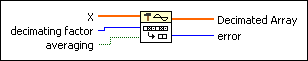X is the input sequence. The number of elements in X must be greater than or equal to the decimating factor.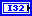decimating factor is the factor by which the VI decimates input sequence X. decimating factor must be greater than zero. The default is 1. If decimating factor is greater than the number of elements in X or less than or equal to zero, this VI sets Decimated Array to an empty array and returns an error.averaging specifies how the VI handles the data points in X. If averaging is FALSE (default), this VI keeps every decimating factor point from X. If averaging is TRUE, each output point in Decimated Array is the mean of the decimating factor input points.Decimated Array returns the decimated sequence of X.error returns any error or warning from the VI. You can wire error to the Error Cluster From Error Code VI to convert the error code or warning into an error cluster.

## Decimate (single shot, CDB)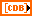X is the complex input sequence for decimation. The number of elements in X must be greater than or equal to the decimating factor.decimating factor is the factor by which the VI decimates input sequence X. decimating factor must be greater than zero. The default is 1. If decimating factor is greater than the number of elements in X or less than or equal to zero, this VI sets Decimated Array to an empty array and returns an error.averaging specifies how the VI handles the data points in X. If averaging is FALSE (default), this VI keeps every decimating factor point from X. If averaging is TRUE, each output point in Decimated Array is the mean of the decimating factor input points.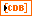Decimated Array returns the decimated sequence of X.error returns any error or warning from the VI. You can wire error to the Error Cluster From Error Code VI to convert the error code or warning into an error cluster.

## Decimate (single shot) Details

If Y represents the output sequence Decimated Array, the Decimate (single shot) VI obtains the elements of the sequence Y using the following equation: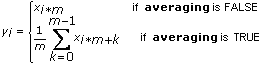for i = 0, 1, 2, …, size – 1,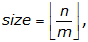where n is the number of elements in X, m is the decimating factor, size is the number of elements in the output sequence Decimated Array, and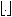gives the largest integer less than or equal to the number.